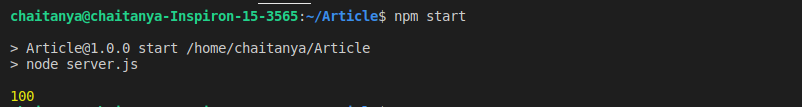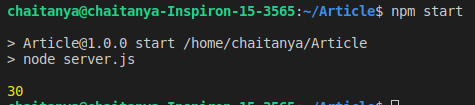# How to write asynchronous function for Node.js ?

The asynchronous function can be written in Node.js using ‘async’ preceding the function name. The asynchronous function returns an implicit Promise as a result. The async function helps to write promise-based code asynchronously via the event loop. Async functions will always return a value. Await function can be used inside the asynchronous function to wait for the promise. This forces the code to wait until the promise returns a result.

Install async from npm in Node.js using the following command:

`npm i async`

Use async inside your Node.js project using require() method.

Example 1: Create an asynchronous function to calculate the square of a number inside Node.js.

• Create a project folder.
• Use the following command to initialize the package.json file inside the project folder.
`npm init -y`
• Install async using the following command:
`npm i async`
• Create a server.js file & write the following code inside it.
• Run the code using the below command
`npm start`

## javascript

 `const async = require(``"async"``);`   `function` `square(x) {` `    ``return` `new` `Promise((resolve) => {` `        ``setTimeout(() => {` `            ``resolve(Math.pow(x, 2));` `        ``}, 2000);` `    ``});` `}`   `async ``function` `output(x) {` `    ``const ans = square(x);` `    ``console.log(ans);` `}`   `output(10);` `const async = require(``"async"``);`   `function` `square(x) {` `    ``return` `new` `Promise((resolve) => {` `        ``setTimeout(() => {` `            ``resolve(Math.pow(x, 2));` `        ``}, 2000);` `    ``});` `}`   `async ``function` `output(x) {` `    ``const ans = await square(x);` `    ``console.log(ans);` `}`   `output(10);`

Output:Example 2: Create an asynchronous function to calculate the sum of two numbers inside Node.js using await. Perform the above procedure to create a Node.js project.

## javascript

 `const async = require(``"async"``);`   `function` `square(a, b) {` `    ``return` `new` `Promise(resolve => {` `        ``setTimeout(() => {` `            ``resolve(a + b);` `        ``}, 2000);` `    ``});` `}`   `async ``function` `output(a, b) {` `    ``const ans = await square(a, b);` `    ``console.log(ans);` `}`   `output(10, 20);`

Output:Whether you're preparing for your first job interview or aiming to upskill in this ever-evolving tech landscape, GeeksforGeeks Courses are your key to success. We provide top-quality content at affordable prices, all geared towards accelerating your growth in a time-bound manner. Join the millions we've already empowered, and we're here to do the same for you. Don't miss out - check it out now!

Previous
Next